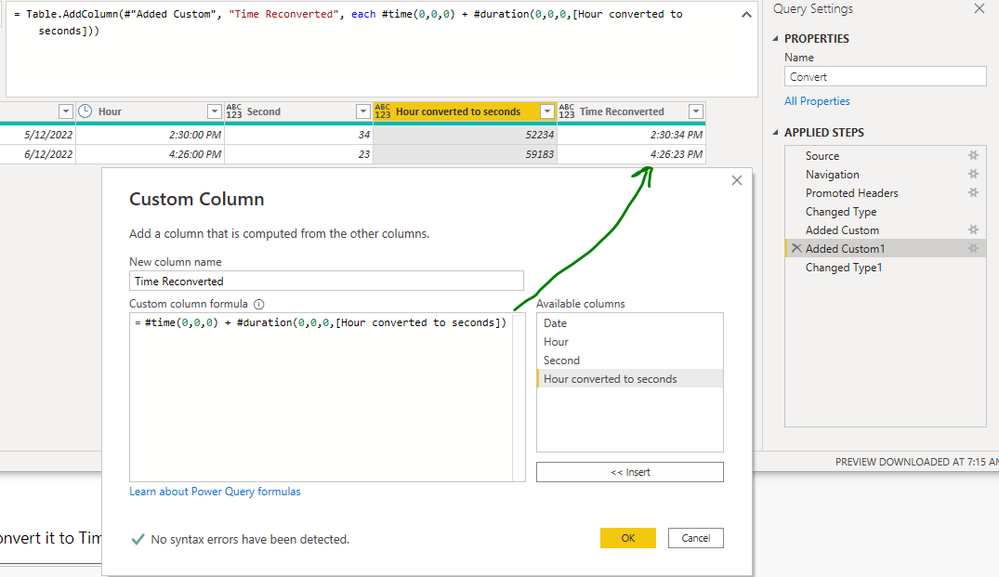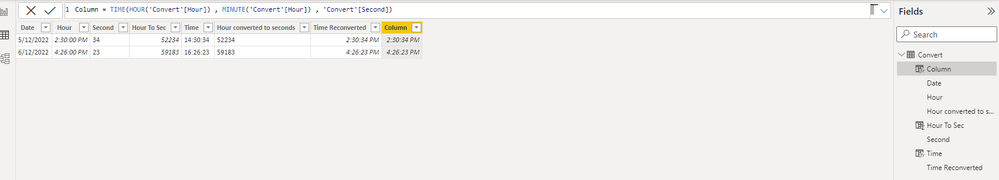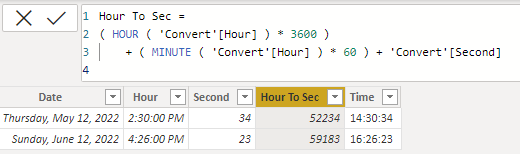cancel
Showing results for
Did you mean:Helper I

## HOW CAN I GET THE FINAL TIME OF THE CALLS?

Hi everyone!

I need to calculate the exact time the calls ended.

I have the following data: date, start time and seconds that the call lasted.

Example: 05/12/22 ; 14:30:00; 34 (sec).

The "Start Time" column is in HOUR format and the column containing seconds is in INTEGER format.

How could I calculate the final time? According to the previous example, it should return the following value: 14:30:34.

Thank you very much. All the best!

1 ACCEPTED SOLUTIONCommunity Champion

Ok lets rather do it in Power Query

Add a custom column to total the secondsHere is the Code :

Time.Hour([Hour]) * 3600
+
Time.Minute([Hour]) * 60
+
[Second]

Then
Add another Custom Column to convert it to Timeand here is the code :

#time(0,0,0) + #duration(0,0,0,[Hour converted to seconds])

Finally Change the type of the column to Time

Third Option would be this DAXLet me know

Regards
Amine Jerbi

and you can follow me on

6 REPLIES 6Community Champion

In Power Query, convert the HOUR column to all Seconds, Add the converted column to the column containing seconds and finaly convert the new column to Time format.

You can do it in DAX as well. The idea is always to render the HOUR column to all seconds...

Regards
Amine Jerbi

and you can follow me onHelper I

I already have the column with all seconds in a integer format. However, I don't know how to convert the column to Time format. I tried it with the function "Convert" but it returns me "dd:mm:yyyy" instead of "hh:nn:ss".

Could you help me? Thank you in advance.

Regards.Community Champion

Hey @BIBITARIFA

Firts add a column to covert the time to all secondsThen add a new column to convert all seconds to TimeAnd here are the 2 DAX codes:

Hour To Sec =
( HOUR ( 'Convert'[Hour] ) * 3600 )
+ ( MINUTE ( 'Convert'[Hour] ) * 60 ) + 'Convert'[Second]

Time =
VAR _Hour =
INT ( MOD ( 'Convert'[Hour To Sec], 86400 ) / 3600 )
VAR _Minute =
DIVIDE (
INT ( MOD ( MOD ( 'Convert'[Hour To Sec], 86400 ), 3600 ) ) - 'Convert'[Second],
60
)
RETURN
_Hour & ":" & _Minute & ":" & 'Convert'[Second]

Regards
Amine Jerbi

and you can follow me onHelper I

Thanks @aj1973 ! I could get the column with the Time. Nevertheless, the format is in Text and I need it in Time. When I try to change the format the result is: #ERROR in all rows. Why I can't convert it to a Time format?

Regards,

Bibi

JaraCommunity Champion

Ok lets rather do it in Power Query

Add a custom column to total the secondsHere is the Code :

Time.Hour([Hour]) * 3600
+
Time.Minute([Hour]) * 60
+
[Second]

Then
Add another Custom Column to convert it to Timeand here is the code :

#time(0,0,0) + #duration(0,0,0,[Hour converted to seconds])

Finally Change the type of the column to Time

Third Option would be this DAXLet me know

Regards
Amine Jerbi

and you can follow me onHelper I

Yes! @aj1973 I finally made it!

I solved it through Power Query. Really, thank you so much for your help. I can't be more grateful.

Kind regards,

Bibi